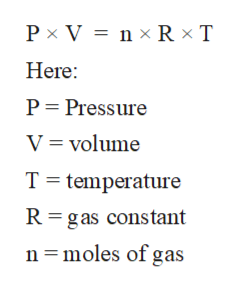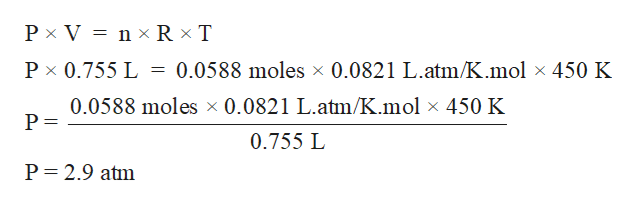# A 755 mL sample of gas which contains 0.0588 moles kept at 450. K would be under what pressure?

Question
1 views

A 755 mL sample of gas which contains 0.0588 moles kept at 450. K would be under what pressure?

check_circle

Step 1

The gaseous state is the state of matter with least intermolecular force of attraction between molecules and highest kinetic energy. The standard properties of gases like Pressure, volume, and temperature can be calculated with the help of ideal gas equation.

Step 2

The ideal gas equation can be written as:help_outlineImage TranscriptioncloseP x V n x RxT Here: P Pressure V volume T temperature R gas constant n moles of gas fullscreen
Step 3

Given:

Volume = 755 mL = 0.755 L

Moles = n = 0.0588 moles

T = 450 K

Substitute the val...help_outlineImage TranscriptioncloseP x V n R x T 0.0588 moles x 0.0821 L.atm/K.mol x 450 K P x 0.755 L 0.0588 moles x 0.0821 L.atm/K.mol x 450 K 0.755 L P 2.9 atm fullscreen

### Want to see the full answer?

See Solution

#### Want to see this answer and more?

Solutions are written by subject experts who are available 24/7. Questions are typically answered within 1 hour.*

See Solution
*Response times may vary by subject and question.
Tagged in

### Ideal gases